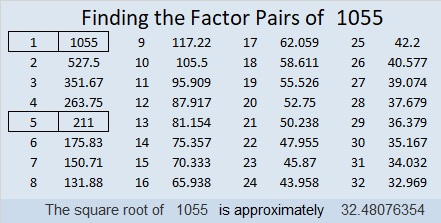# 1055 and Level 1

I bet you can solve this puzzle like clockwork!Print the puzzles or type the solution in this excel file: 10-factors-1054-1062

Here is a little bit about the number 1055:

• 1055 is a composite number.
• Prime factorization: 1055 = 5 × 211
• The exponents in the prime factorization are 1 and 1. Adding one to each and multiplying we get (1 + 1)(1 + 1) = 2 × 2 = 4. Therefore 1055 has exactly 4 factors.
• Factors of 1055: 1, 5, 211, 1055
• Factor pairs: 1055 = 1 × 1055 or 5 × 211
• 1055 has no square factors that allow its square root to be simplified. √1055 ≈ 32.48076This site uses Akismet to reduce spam. Learn how your comment data is processed.